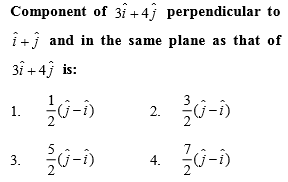Complete Question Bank + Test Series
Complete Question Bank

Difficulty Level: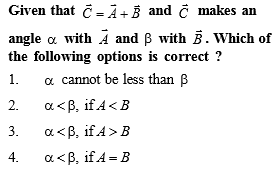Complete Question Bank + Test Series
Complete Question Bank

Difficulty Level: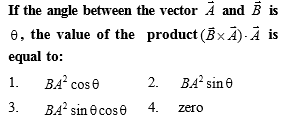Complete Question Bank + Test Series
Complete Question Bank

Difficulty Level: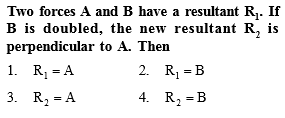Complete Question Bank + Test Series
Complete Question Bank

Difficulty Level:

Two forces are such that the sum of their magnitudes is 18 N and their resultant is perpendicular to the smaller force and magnitude of resultant is 12 N. Then the magnitudes of the forces are

(1) 12 N, 6 N

(2) 13 N, 5N

(3) 10 N, 8 N

(4) 16 N, 2 N

Complete Question Bank + Test Series
Complete Question Bank

Difficulty Level:

If the magnitude of sum of two vectors is equal to the magnitude of difference of the two vectors, the angle between these vectors is
(a)90° (b)45° (c) 180° (d) 0°

Complete Question Bank + Test Series
Complete Question Bank

Difficulty Level:

Six vector  have the directions indicated in the figure.Which of the following statements may be true?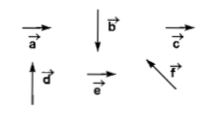(a) $\stackrel{\to }{b}+\stackrel{\to }{c}=\stackrel{\to }{-f}$                        (b) $\stackrel{\to }{d}+\stackrel{\to }{c}=\stackrel{\to }{f}$

(c) $\stackrel{\to }{d}+\stackrel{\to }{e}=\stackrel{\to }{f}$                        (d) $\stackrel{\to }{b}+\stackrel{\to }{e}=\stackrel{\to }{f}$

Complete Question Bank + Test Series
Complete Question Bank

Difficulty Level:

In the given figure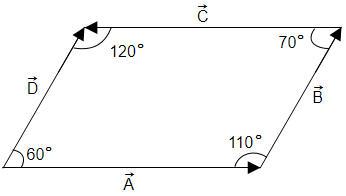(A)  Angle between $\stackrel{\to }{\mathrm{A}}$ and $\stackrel{\to }{\mathrm{B}}$ is $110°$

(B)  Angle between $\stackrel{\to }{\mathrm{C}}$ and $\stackrel{\to }{\mathrm{D}}$ is $60°$

(C)  Angle between $\stackrel{\to }{\mathrm{B}}$ and $\stackrel{\to }{\mathrm{C}}$ is $110°$

(D)  Angle between $\stackrel{\to }{\mathrm{B}}$ and $\stackrel{\to }{\mathrm{C}}$ is $70°$

Complete Question Bank + Test Series
Complete Question Bank

Difficulty Level:

A force of 6 N and another of 8 N can be applied together to produce the effect of a single force of -

(A)  1 N

(B)  11 N

(C)  15 N

(D)  20 N

Complete Question Bank + Test Series
Complete Question Bank

Difficulty Level:

Which of the sets given below may represent the magnitude of resultant of three vectors adding to zero?

(A)  2, 4, 8

(B)  4, 8, 16

(C)  1, 2, 1

(D)  0.5, 1, 2